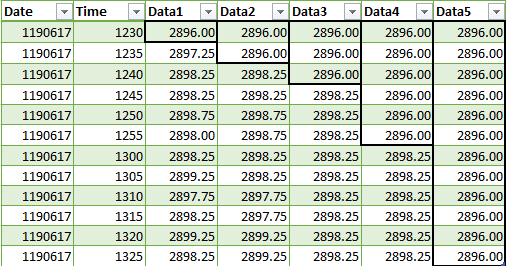# Multi-Time Frame – Using Built-in Indicators and Multi Data Charts

A reader of this blog wanted to be able to use different time frames and some built-in indicators and output the information in a similar fashion as I did in the original MTF post.  There are numerous ways to program this but the two easiest are to use data structures such as arrays or vectors or use TradeStation’s own multi data inputs.  The more complicated of the two would be to use arrays and stay compliant with Multicharts.  Or in that same vein use vectors and not stay compliant with Multicharts.  I chose, for this post, the down and dirty yet compliant method.  [NOTE HERE! When I started this post I didn’t realize it was going to take the turn I ended up with.  Read thoroughly before playing around with the code to see that it is what you are really, really looking for.]  I created a multi data chart with five-time frames: 5,10,15,30 and 60 minutes.  I then hid data2 thru data5.  I created an MTF indicator that plots the relationship of the five time frames applied to the ADX indicator with length 14.  If the ADX > 20 then the plot will be green else it will be red.  If all plots align, then the composite plot will reflect the alignment color.

``````{EasyLanguage MultiTime Frame Indicator)
written by George Pruitt - copyright 2019 by George Pruitt
}

If barNumber > 1 then
Begin

If condition10 then setPlotColor(1,Green) else SetPlotColor(1,Red);
If condition11 then setPlotColor(2,Green) else SetPlotColor(2,Red);
If condition12 then setPlotColor(3,Green) else SetPlotColor(3,Red);
If condition13 then setPlotColor(4,Green) else SetPlotColor(4,Red);
If condition14 then setPlotColor(5,Green) else SetPlotColor(5,Red);

condition6 = condition10 and condition11 and condition12 and condition13 and condition14;
Condition7 = not(condition10) and not(condition11) and not(condition12) and not(condition13) and not(condition14);

If condition6 then setPlotColor(7,Green);
If condition7 then setPlotColor(7,Red);

If condition6 or condition7 then plot7(7,"trend");

Plot6(5,"line");
Plot1(4,"t1");
Plot2(3,"t2");
Plot3(2,"t3");
Plot4(1,"t4");
Plot5(0,"t5");

end;``````
MTF with 5 data streams and ADX

This code is very similar to the original MTF indicator, but here I simply pass a pointer to the different time frames to the ADX function.  Since the ADX function only requires a length input I had assumed I could use the following format to get the result for each individual time frame: